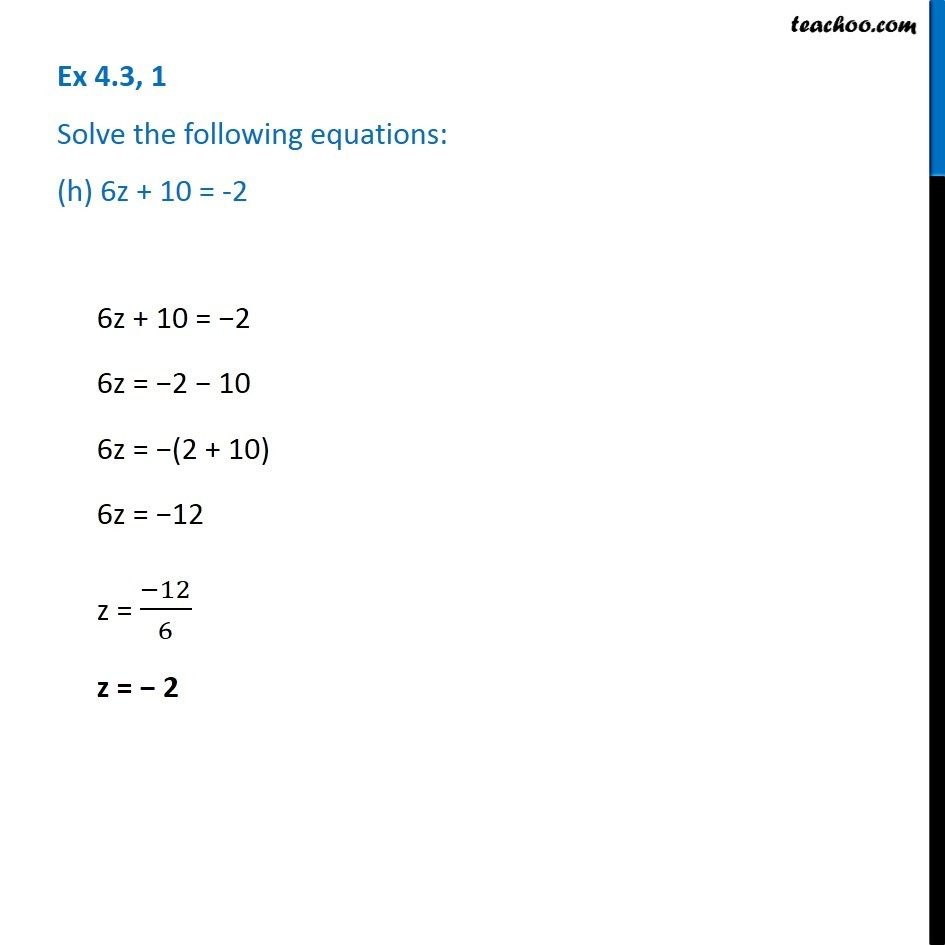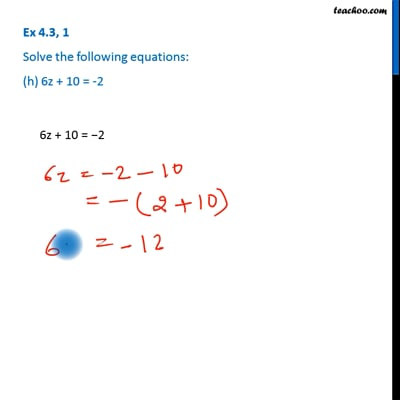Ex 4.3

Chapter 4 Class 7 Simple Equations
Serial order wiseThis video is only available for Teachoo black users

Get live Maths 1-on-1 Classs - Class 6 to 12

### Transcript

Ex 4.3, 1 Solve the following equations: (h) 6z + 10 = -26z + 10 = −2 6z = −2 − 10 6z = −(2 + 10) 6z = −12 z = (−12)/6 z = − 2